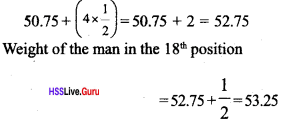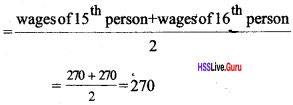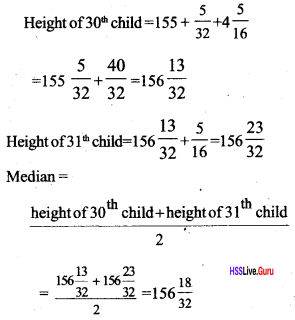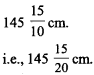# Kerala Syllabus 10th Standard Maths Solutions Chapter 11 Statistics

## Kerala State Syllabus 10th Standard Maths Solutions Chapter 11 Statistics

### Statistics Textbook Questions & Answers

Textbook Page No. 245

Statistics Class 10 Kerala Syllabus Questions 1.
The distance covered by an athlete in long jump practice are
6.10, 6.20, 6.18, 6.20, 6.25, 6.21, 6.15, 6.10
in meters. Find the mean and median. Why is it that there is not much difference between these?
Mean = $$\begin{array}{l}{=\frac{6.10+6.20+6.18+6.20+6.25+6.21+6.15+6.10}{8}} \\ {=\frac{49.39}{8}=6.17}\end{array}$$
If distances are written in ascending order
6.10, 6.15, 6.18, 6.20, 6.21, 6.25
Median = $$\frac{6.18+6.20}{2}=6.19 \mathrm{m}$$
Mean and median are gives the average dis¬tance covered by a person. Hence they will not have much difference..

Polynomial in Descending Order Calculator is a free online tool that determines the descending order of a polynomial in just a few taps.

Sslc Maths Statistics Kerala Syllabus Questions 2.
The table below gives the rainfall during one week of September 2015 in various districts of Kerala.

 District Rainfall (mm) Kasaragod 66.7 Kannur 56.9 Kozhikode 33-5 Wayanad 20.5 Malappuram 13-5 Palakkad 56.9 Thrissur 53-4 Ernakulam 70.6 Kottayam 50.3 Idukki 30.5 Pathanamthitta 56.4 Alapuzha 45-5 Kollam 56.3 Thiruvananthapuram 89.0

Calculate the mean and median rainfall in Kerala during this week. Why is the mean less than median?
Mean = Total amount of rain / No. of districts
= $$\frac { 700 }{ 14 }$$ = 50
In ascending order:
13.5, 20.5, 30.5, 33.5, 45.5, 50.3, 53.4, 56.3, 56.4, 56.9, 56.9, 66.7, 70.6, 89
Median $$=\frac{53.4+56.3}{2}=54.85$$
The mean is less than median because the number contains are far small and large numbers than mean.

Use this number sequence calculator to easily calculate the n-th term of an arithmetic, geometric or fibonacci sequence.

Sslc Statistics Solutions Kerala Syllabus Questions 3.
Prove that for a set of numbers arithmetic sequence, the mean and median are equal.
Let a, a+d, a+3d, a+4d are the numbers of an arithmetic sequence, then median = $$\frac{a+a+4 d}{2}=\frac{2 a+4 d}{2}=a+2 d$$
Median will be the term which is at center = a + 2d
∴ A set of numbers in arithmetic sequence, the mean and median are equal.

Textbook Page No. 248

Sslc Maths Statistics Notes Kerala Syllabus  Questions 1.
35 households in a neighborhood are sorted according to their monthly income in the table below.

 Monthly income (Rs) Number of households 4000 3 5000 7 6000 8 7000 5 8000 5 9000 4 10000 3

Calculate the median income.

 Monthly income (Rs) Number of households up to 4000 3 up to5000 10 up to 6000 18 up to 7000 23 up to 8000 28 up to 9000 32 up to10000 35

In the table monthly income up to 18th place be 6000 rupees.
That is 18th place family also includes in the middle of total number of families.
∴ Median of the income = Rs. 6000

Sslc Maths Chapter 11 Statistics Kerala Syllabus Questions 2.
The table below shows the workers in a factory sorted according to their daily wages.

 Daily wages (Rs) Number of workers 400 2 500 4 600 5 700 7 800 5 900 4 1000 3

Calculate the median daily wage.

 Daily wages (Rs) Number of workers up to 400 2 up to 500 6 up to 600 11 up to 700 18 up to 800 23 up to 900 27 up to 1000 30

Total number of workers = 30
Half= 15
So median is the wage of 15th worker.
The daily wages between the place 11 and 18 = 700 rupees
Median of daily wages = 700 rupees

Sslc Maths Chapter 11 Kerala Syllabus Questions 3.
The table below gives the number of babies born in a hospital during a week, sorted according to their birth weight.

 Weight (kg) Number of babies 2.500 4 2.600 6 2.750 8 2.800 10 3.000 12 3-i5o 10 3-250 8 3-300 7 3-5°o 5

Calculate the median birth-weight

 Weight (kg) Number of babies up to 2.500 4 up to 2.600 10 up to 2.750 18 up to 2.800 28 up to 3.000 40 up to 3.150 50 up to 3.250 58 up to 3.300 65 up to 3.500 70

Total number of babies = 70
Half =35
So median is the weight of 35th baby.
The weight of 35 111 child be in between 29 and 40 placed child, its weight will be 3 kg.
∴ Median of the weight = 3 kg.

Textbook Page No. 254

Sslc Statistics Notes Kerala Syllabus Questions 1.
The table shows some households sorted according to their usage of electricity:

 Electricity usage (units) Number of households 80 – 90 3 90 – 100 6 100 – 110 7 110 – 120 10 120 – 130 9 130 – 140 4

Calculate the median usage of electricity.

 Usage of electricity (units) Number of households less than 90 3 less than 100 9 less than 110 16 less than 120 26 less than 130 35 less than 140 39

Half of the number of houses = 20
We have to find the electricity usage of the 20th house. According to this, we can divide

Statistics Class 10 State Syllabus Question 2.

 Weight Number less than 45.5 5 less than 50.5 12 less than 55.5 22 less than 60.5 30 less than 65.5 34

Total number of children = 34
It is an even number, so we will take the half of sum of weight of the children, those are in the 17 and 18 positions. According to this child between 13 and 22 have weight between 50.5 and 55.5. Our required children (between and 17 and 18) are in these positions. Divide 5 years from 50.5 to 55.5 into 10 equal, parts. Let consider each part have one child.

Weight of each part = $$\frac { 5 }{ 10 }$$ = $$\frac { 1 }{ 2 }$$.
Hence weight of a child in 13th place is in middle of 50.5 and 51. That is 50.75. Further, each student’s weight is increased by 5.
Hence weight of the man in the 17th positionMedian $$=\frac{52.75+53.25}{2}=53$$

Statistics 10th Standard State Syllabus Question 3.
The workers of a company are arranged as given below. Calculate median

 Income (Rs) Number of workers 450 2 500 3 550 5 600 8 650 6 700 5 750 1

 Income (Rs) Number of workers up to 450 2 up to 500 5 up to 550 10 up to 600 18 up to 650 24 up to 700 29 up to 750 30

Total number of workers = 30
Half = 15
So median is the wage of 15th worker.
The daily wage between the place 10 and 18 = 600 rupees
Median of daily wage = 600 rupees

### Statistics SCERT Questions & Answers

Scert Class 10 Maths Statistics Kerala Syllabus Question 5.
10 households in a neighborhood are sorted according to their monthly income are given below
16500, 21700, 18600, 21050, 19500, 17000, 21000, 18000, 22000, 75000
a. What is the mean income of these 10 families?
b. How many families have monthly incomes less than the mean income? Prove that in such situation this average is suitable or not?
a. Mean = $$\frac { sum }{ number }$$ = $$\frac { 248000 }{ 10 }$$ = 24800
b. 9 families have monthly income less than the mean income. So in this situation this is not a suitable average.

Sslc Maths Statistics Questions And Answers Kerala Syllabus Question 6.
Number of members in 10 families, collected by mathematics club survey are given. Calculate mean; median and explain. Which is the suitable average?.
4, 2, 3, 5, 4, 3, 2, 20, 4, 3
a. Mean = 5
b. Median = 3.5
Suitable average median = 3.5

Sslc Statistics Questions Kerala Syllabus Question 7.
Weekly Wages of 9 persons Working in a factory are given. Find the median 2100; 3500, 2100, 2500, 2800, 4900, 2300, 2200, 3300
Write the number in order.
2100, 2100, 2200, 2300, 2500, 2800, 3300, 3300, 3500 (1)

Median = 2500

Statistics Class 10 State Board Kerala Syllabus Question 8.
The table shows the workers doing different jobs in a factory according to their daily wages.

 Daily wages(Rs) Number of workers 225 4 250 7 270 9 300 5 350 3 400 2

Calculate median of daily wages.

 Daily wages(Rs) Number of workers up to 225 4 up to 250 11 up to 270 20 up to 300 25 up to 350 28 upto400 30

The worker in the 12th position to 20th position has daily wage 270 ie, MedianSslc Maths Chapter 11 Solutions Kerala Syllabus Question 9.
The table below shows the 60 children in a class sorted according to their heights

 Height (cm) Number of children’s 140-145 5 145-150 8 150-155 12 155-160 16 160-165 11 165-170 5 170-175 3

Find the median height?

 Height (cm) Number of children’s Belowl45 5 Below 150 13 Below 155 25 Below 160 41 Below 165 52 Below 170 57 Below 175 60 1Sslc Maths Chapter Statistics Kerala Syllabus  Question 10.
a. 100
b. 25
c.

 Mid value (x) No.of Workers (y) x, y 130 16 2080 150 11 1650 170 20 3400 190 28 5320 210 18 3780 230 7 1610

Mean = $$\frac { 17840 }{ 100 }$$ = 178.4

Hss Live Guru 10th Maths Kerala Syllabus Question 11.
The mean of the frequency table given below is 50. Then find out the values of a and b.mean = 50
∴ $$\frac{3480+30 a+70 b}{120}=50$$
30a + 70b = 6000 – 3480
30a + 70b = 2520 (1)
17 + 32 + 19 + a + b = 120
68 + a + b = 120,
a + b = 52 (2)
(2) x 30 => 30a + 30b = 1560
30a + 70b = 2520,
30a + 30b = 1560,
40b = 960,
b = $$\frac { 960 }{ 40 }$$ = 24,
a + 24 = 52
a = 52 – 24 = 28,
∴ a = 28, b = 24

Long Answer Type Questions (Score 5)

10th Standard Maths Statistics Kerala Syllabus Question 21.
The table below shows groups of children in a class according to their heights:

 Height (cm) Number of children 135-140 5 140-145 8 145 – 150 10 150-155 9 155-160 6 160-165 3

a. If the children are lined up according to their heights, the median is the height of the child in which position?
b. According to the table, the height of this child is between what limits?
c. What are the assumptions used to compute the median?
d. What is the median height according to these assumptions?

 Height (cm) Number of children Below 140 5 Below 145 13 Below 150 23 Below 155 32 Below 160 38 Below 165 41

a. Height of the 21st child is the median height.
b. Height of the 21st child is between 145 cm and 150 cm.
c. Methods to find the median are.
1. Divide 5cm in between 145 cm and 150 cm into 10 equal sections.
2. Consider that the height of each sub-group is exactly on the midpoint of the subgroup.
Height of the 14th child is in between 145 cm and 145 $$\frac { 5 }{ 10 }$$ cm.
i.e., 145 $$\frac { 5 }{ 20 }$$ cm.
Similarly, the height of the 15th student is in between 145 $$\frac { 5 }{ 10 }$$ cm andHence height of each child can be increased by $$\frac { 5 }{ 10 }$$ cm.
There are 7 children to reach the 21st child from 14th child.
i.e., 14 th term is 145 $$\frac { 5 }{ 20 }$$ and common difference is $$\frac { 5 }{ 10 }$$.
Mean is the 21 st term of the arithmetic sequence.Use our simple online Limit Of Sequence Calculator to find the Limit with step-by-step explanation.

### Statistics Menton Map

Arithmetic mean is the sum divided by the number of terms.

Mean = $$\frac { sum of terms }{ Number of terms }$$

When the numbers are arranged in a ascending order, then the middle term is the median.
i.e., half of the total frequency will give the median.